Open in App
Not now

# Convert list-like column elements to separate rows in Pandas

• Last Updated : 12 Nov, 2020

A dataframe is a tabular structure where data is arranged in rows and columns. Often while working with real data, columns having list-like elements are encountered. List-like means that the elements are of a form that can be easily converted into a list. In this article, we will see various approaches to convert list-like column elements to separate rows.

First, let us create a data frame which we will use for all the approaches.

## Python

 `# import Pandas library``import` `pandas as pd`` ` `# create dataframe with a column (names) having list-like elements``data ``=` `{``'id'``: [``1``, ``2``, ``3``],``        ``'names'``: [``"Tom,Rick,Hardy"``, ``"Ritu,Shalini,Anjana"``, ``"Ali,Amir"``]}`` ` `df ``=` `pd.DataFrame(data)`` ` `print``(df)`

Output: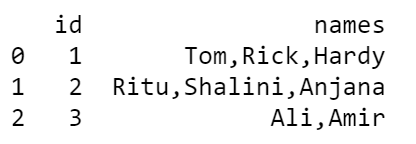### Now, let us explore the approaches step by step.

Method 1: Using Pandas melt function

First, convert each string of names to a list.

## Python

 `# assign the names series to a variable with ``# the same name and create a list column``df_melt ``=` `df.assign(names``=``df.names.``str``.split(``","``))`` ` `print``(df_melt)`

Output: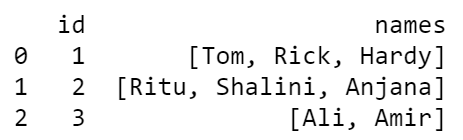Now, split names column list values (columns with individual list values are created).

## Python

 `df_melt.names.``apply``(pd.Series)`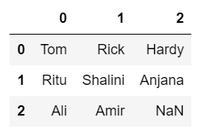Merge the new columns with the rest of the data set.

## Python

 `df_melt.names.``apply``(pd.Series) \``    ``.merge(df_melt, right_index ``=` `True``, left_index ``=` `True``)`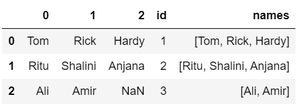Drop the old names list column and then transform the new columns into separate rows using the melt function.

## Python

 `df_melt.names.``apply``(pd.Series) \``   ``.merge(df_melt, right_index ``=` `True``, left_index ``=` `True``) \``   ``.drop([``"names"``], axis ``=` `1``) \``   ``.melt(id_vars ``=` `[``'id'``], value_name ``=` `"names"``)`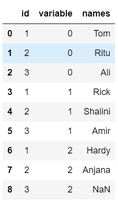Now combine the above steps. Also, an additional column ‘variable’ containing the ids of the numeric columns is seen. This column is dropped and empty values are removed.

## Python

 `df_melt ``=` `df.assign(names``=``df.names.``str``.split(``","``))``df_melt ``=` `df_melt.names.``apply``(pd.Series) \``    ``.merge(df_melt, right_index``=``True``, left_index``=``True``) \``    ``.drop([``"names"``], axis``=``1``) \``    ``.melt(id_vars``=``[``'id'``], value_name``=``"names"``) \``    ``.drop(``"variable"``, axis``=``1``) \``    ``.dropna()`` ` `print``(df_melt)`

Output: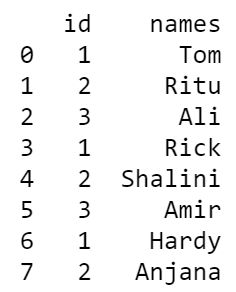Method 2: Using Pandas stack function

Convert each string of names to a list then use the pandas stack() function for pivoting the columns to index.

## Python

 `# convert names series into string using str method``# split the string on basis of comma delimiter``# convert the series into list using to_list method``# use stack to finally convert list elements to rows`` ` `df_stack ``=` `pd.DataFrame(df.names.``str``.split(``","``).to_list(), index``=``df.``id``).stack()``df_stack ``=` `df_stack.reset_index([``"id"``])``df_stack.columns ``=` `[``"id"``, ``"names"``]`` ` `print``(df_stack)`

Output: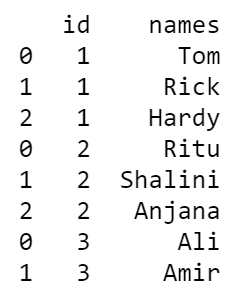Method 3: Using Pandas explode function

Convert each string of names to a list and use Pandas explode() function to split the list by each element and create a new row for each of them.

## Python

 `# use explode to convert list elements to rows``df_explode ``=` `df.assign(names``=``df.names.``str``.split(``","``)).explode(``'names'``)`` ` `print``(df_explode)`

Output: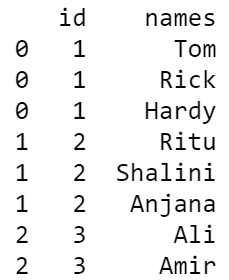My Personal Notes arrow_drop_up